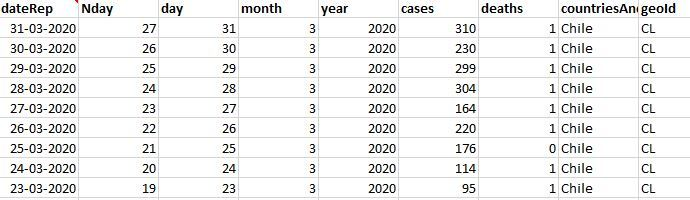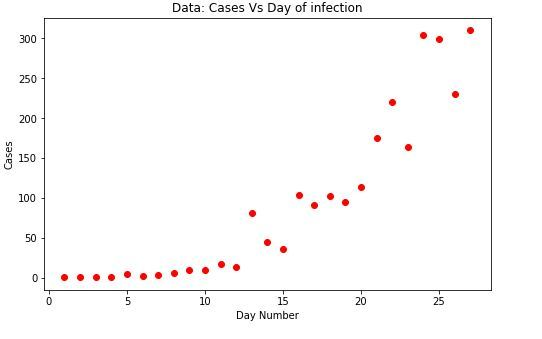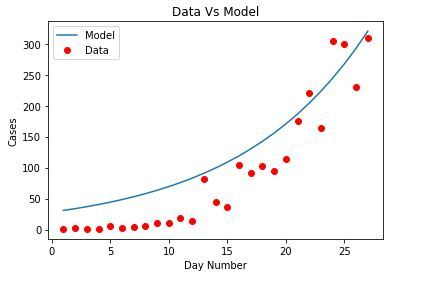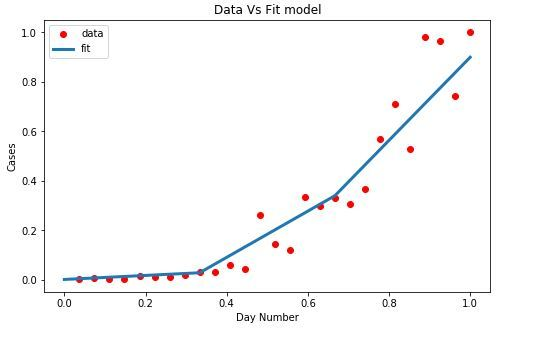Related Articles
COVID-19 Peak Prediction using Logistic Function
• Difficulty Level : Medium
• Last Updated : 30 Jun, 2020

Making fast and accurate decisions are vital these days and especially now when the world is facing such a phenomenon as COVID-19, therefore, counting on current as well as projected information is decisive for this process.
In this matter, we have applied a model in which is possible to observe the peak in specific country cases, using current statistical information, hoping it can be used as foundation support to take action in this scenario. To accomplish this objective, Non-linear regression has been applied to the model, using a logistic function. This process consists of:

• Data Cleaning
• Choosing the most suitable equation which can be graphically adapted to the data, in this case, Logistic Function (Sigmoid)
• Database Normalization
• Fitting of the model to our dataset using “curve_fit” process, obtaining new reference beta.
• Model evaluation

Dataset is public, and it is available at Data.europa.eu following this link: DATASET

Data Cleaning: The data available has been originally labelled. We were able to identify two countries which did not mention geographical location, this information was added however it wouldn´t contribute to the model significantly. A new column is added to the dataset named “n-day” to show the consecutive number of days.

Code: Importing Libraries

 `# import libraries``import` `pandas as pd ``import` `numpy as np ``import` `matplotlib.pyplot as plt ``%` `matplotlib inline `` ` `# sklearn specific function to obtain R2 calculations ``from` `sklearn.metrics ``import` `r2_score `

Code: Usign data

 `# Data Reading``df ``=` `pd.read_excel(``"C:/BaseDato / COVID-19-310302020chi.xlsx"``)``df.head()`

Output:Code:

 `# Initial Data Graphics``plt.figure(figsize ``=``(``8``, ``5``))`` ` `x_data, y_data ``=` `(df[``"Nday"``].values, df[``"cases"``].values)`` ` `plt.plot(x_data, y_data, ``'ro'``)``plt.title(``'Data: Cases Vs Day of infection'``)``plt.ylabel(``'Cases'``)``plt.xlabel(``'Day Number'``)`

Output:Code: Choosing the model

We apply logistic function, a specific case of sigmoid functions, considering that the original curve starts with slow growth remaining nearly flat for a time before increasing, eventually it could descend or maintain its growth in the way of an exponential curve.
The formula for the logistic function is:

`Y = 1/(1+e^B1(X-B2))`

Code: Construction of the model

 `# Definition of the logistic function``def` `sigmoid(x, Beta_1, Beta_2):``     ``y ``=` `1` `/` `(``1` `+` `np.exp(``-``Beta_1``*``(x``-``Beta_2)))``     ``return` `y`` ` `# Choosing initial arbitrary beta parameters``beta_1 ``=` `0.09``beta_2 ``=` `305`` ` `# application of the logistic function using beta ``Y_pred ``=` `sigmoid(x_data, beta_1, beta_2)`` ` `# point prediction``plt.plot(x_data, Y_pred ``*` `15000000000000.``, label ``=` `"Model"``)``plt.plot(x_data, y_data, ``'ro'``, label ``=` `"Data"``)``plt.title(``'Data Vs Model'``)``plt.legend(loc ``=``'best'``)``plt.ylabel(``'Cases'``)``plt.xlabel(``'Day Number'``)`

Output:Data Normalization: Here, variables x and y are normalized assigning them a 0 to 1 range (depending on each case). So both can be interpreted in equal relevance.
Reference – information

Code:

 `xdata ``=` `x_data ``/` `max``(x_data)``ydata ``=` `y_data ``/` `max``(y_data)`

Model Fitting:
The objective is to obtain new B optimal parameters, to adjust the model to our data. We use “curve_fit” which uses non-linear least squares to fit the sigmoid function. Being “popt” our optimized parameters.

Code: Input

 `from` `scipy.optimize ``import` `curve_fit``popt, pcov ``=` `curve_fit(sigmoid, xdata, data)`` ` `# imprimir los parámetros finales``print``(``" beta_1 = % f, beta_2 = % f"` `%` `(popt[``0``], popt[``1``]))`

Output:

`beta_1 = 9.833364, beta_2 = 0.777140`

Code: New Beta values are applied to the model

 `x ``=` `np.linspace(``0``, ``40``, ``4``)``x ``=` `x ``/` `max``(x)`` ` `plt.figure(figsize ``=` `(``8``, ``5``))`` ` `y ``=` `sigmoid(x, ``*``popt)`` ` `plt.plot(xdata, ydata, ``'ro'``, label ``=``'data'``)``plt.plot(x, y, linewidth ``=` `3.0``, label ``=``'fit'``)``plt.title(``"Data Vs Fit model"``)``plt.legend(loc ``=``'best'``)``plt.ylabel(``'Cases'``)``plt.xlabel(``'Day Number'``)``plt.show()`Model Evaluation: The model is ready to be evaluated. The data is split in at 80:20, for training and testing respectively. The data is applied to the model obtaining the corresponding statistical means to evaluate the distance of the resulting data from the regression line.

Code: Input

 `# Model accuracy calculation``# Splitting training and testing data`` ` `L ``=` `np.random.rand(``len``(df)) < ``0.8` `# 80 % training data``train_x ``=` `xdata[L]``test_x ``=` `xdata[~L]``train_y ``=` `ydata[L]``test_y ``=` `ydata[~L]`` ` `# Construction of the model ``popt, pcov ``=` `curve_fit(sigmoid, train_x, train_y)`` ` `# Predicting using testing model``y_predic ``=` `sigmoid(test_x, ``*``popt)`` ` `# Evaluation``print``(``"Mean Absolute Error: %.2f"` `%` `np.mean(np.absolute(y_predic ``-` `test_y)))``print``(``"Mean Square Error (MSE): %.2f"` `%` `np.mean(( test_y ``-` `y_predic)``*``*``2``))``print``(``"R2-score: %.2f"` `%` `r2_score(y_predic, test_y))`

Output:

```Mean Absolute Error: 0.06
Mean Square Error (MSE): 0.01
R2-score: 0.93```My Personal Notes arrow_drop_up